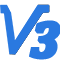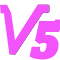一、从牛顿大炮说起

牛顿曾说在一座高山上架起一门大炮，只要这门炮的威力足够大，炮弹的速度足够快，炮弹就可以围绕地球不停的转而不会掉下来。那么对于一枚火箭，速度多快才能入轨？中学课本告诉我们，只要达到第一宇宙速度7.9km/s，我们的火箭就能够进入地球轨道。

(1) 速度大小必须达到7.9千米每秒吗？

(2) 对速度方向没有要求吗？

(3) 轨道的形状怎么确定？

(4) 怎么控制火箭进入预定的轨道?

牛顿大炮的例子中，我们可以感觉到以上问题的答案。大炮在山顶的位置、炮弹的出膛速度、以及速度的角度，共同决定了炮弹的轨道的形状。下面我们就来谈谈这些问题。

(1) 垂直起飞。发动机点火，待发动机推力稳定后，放开火箭。火箭离开发射塔，快速穿过稠密的底层大气层。

(2) 程序转弯。启动转弯过程，以适当的角度穿越跨声速段，防止箭体结构破坏。同时使得火箭在关机点达到所需的速度方向。

(3) 主动段关机。保持速度方向，不断加速，当速度达到所需速度时，执行发动机关机指令。

(4) 星箭分离。分离卫星和火箭，启动反推火箭，让火箭刹车，以免碰撞卫星。同时使火箭减速落回地面。

(5) 入轨。卫星进入轨道。

其中（1）（2）（3）并称为主动段，（4）（5）称为被动段。火箭入轨后的参数唯一取决于主动段关机点的参数。即火箭从发射架上起飞，飞到某个位置然后执行发动机关机指令。在关机点的位置、速度、速度方向就唯一确定了其入轨后的轨道参数。

如图3个关机点参数为：到地心的距离$r_k$、速度大小$V_k$、弹道倾角$\Theta _{k}$。那么如何根据想要的卫星的轨道参数，确定所需要的主动段关机点参数呢?

[修改于 3 年前 - 2019-07-27 03:44:00]

17

42年10个月前 修改于 2年10个月前

三、由轨道参数计算关机点参数

中学物理就讲过，卫星的轨道形状是一个椭圆。地球在椭圆的一个焦点上，而椭圆的参数包括半长轴a、偏心率e。

在研究轨道问题时，常常在极坐标系下进行，如下图所示。

图中，$p$为近地点，$a$为远地点。已知轨道近地点到地心的距离$r_a$，远地点到地心的距离$r_p$，近地点的位置$p$，关机点与地心连线与$op$的夹角为$f_k$。那么关机点参数可以用下面的公式进行计算。

$$r_k=\frac{p}{1+e\cdot cosf}$$

$$V_k=\sqrt{\frac{\mu }{p}(1+2\cdot e \cdot cosf+e^2))}$$

$$tan\Theta =\frac{e\cdot sinf}{1+e\cdot cosf}$$

$\mu$为地球引力常数，$\mu=3.986\times 10^{14}$，

$e$为椭圆轨道偏心率,$e=\frac{r_a-r_p}{r_a+r_p}$,

$a$为椭圆半长轴,$a=\frac{r_a+r_p}{2}$,

$p$为椭圆半通径，$p=a\cdot (1-e^2)$。

（1）关机点速度应小于第二宇宙速度，因为大于第二宇宙速度的话就飞离地球了。

（2）弹道倾角应在0~90（不包括90度）度之间，$\Theta=90^{\circ}$垂直向上发射火箭一定会落下来。

（3）椭圆轨道不应与地面有交点。因为砸到人不好。

1

##### 200字以内，仅用于支线交流，主线讨论请采用回复功能。2年10个月前 修改于 1年11个月前

打算发射一枚火箭入轨，轨道参数是这样的。在近地点到地面的高度为100km，远地点到地面的高度为200km，关机点和近地点的夹角是120度。已知地球平均半径R=6371km，问火箭主动段关机点的参数？

$$r_a=6371+200=6571km$$

$$r_p=6371+100=6471km$$

$$e=\frac{r_a-r_p}{r_a+r_p}=0.00767$$

$$a=\frac{r_a+r_p}{2}=6531km$$

$$p=a\cdot (1-e^2)=6520.62km$$

$$r_k=\frac{p}{1+e\cdot cosf}=6545.72km$$

$$h_k=r_k-R=174.72km$$

$$V_k=\sqrt{\frac{\mu }{p}(1+2\cdot e \cdot cosf+e^2))}=7.79km/s$$

$$tan\Theta =\frac{e\cdot sinf}{1+e\cdot cosf}=6.67/time 10^{-3}，\Theta=0.38^{\circ}$$

(1)火箭入轨后的轨道唯一取决于主动段关机点的参数。

(2)可以根据椭圆轨道的参数计算出关机点的参数。

(3)通过设计火箭主动段弹道，即可实现火箭进入预定轨道。

(4)控制的过程是，（a）首先火箭垂直起飞，以最快速度离开稠密的底层大气，减少能量消耗。（b）然后进行程序转弯，在跨声速段，保持攻角为零，避免火箭受气动力过大导致结构破坏，最后要转弯到指定的弹道倾角，并保持这个角度。（c）当火箭加速到关机速度时，执行关机指令，发动机关机。启动反推火箭，星箭分离，卫星进入轨道。

(5)注意设计的椭圆轨道不应与地面有交点，会变成弹道导弹的，会砸到人的

END

2020/06/20 18:41 修改：补一个仿真结果

1

##### 200字以内，仅用于支线交流，主线讨论请采用回复功能。2年10个月前

##### 200字以内，仅用于支线交流，主线讨论请采用回复功能。2年10个月前

##### 200字以内，仅用于支线交流，主线讨论请采用回复功能。Robot_V12年10个月前

##### 200字以内，仅用于支线交流，主线讨论请采用回复功能。3141522年10个月前

##### 200字以内，仅用于支线交流，主线讨论请采用回复功能。2年10个月前

##### 200字以内，仅用于支线交流，主线讨论请采用回复功能。dkhtt2年10个月前

##### 200字以内，仅用于支线交流，主线讨论请采用回复功能。zx-865042年0个月前

2

##### 200字以内，仅用于支线交流，主线讨论请采用回复功能。JoeyBoy9个月9天前

##### 200字以内，仅用于支线交流，主线讨论请采用回复功能。JoeyBoy9个月7天前

Wolfram Mathematica 12计算结果：

##### 200字以内，仅用于支线交流，主线讨论请采用回复功能。9个月7天前

##### 200字以内，仅用于支线交流，主线讨论请采用回复功能。JoeyBoy9个月7天前

##### 200字以内，仅用于支线交流，主线讨论请采用回复功能。JoeyBoy9个月7天前

##### 200字以内，仅用于支线交流，主线讨论请采用回复功能。TonyCheng9个月7天前

##### 200字以内，仅用于支线交流，主线讨论请采用回复功能。JoeyBoy9个月6天前

##### 200字以内，仅用于支线交流，主线讨论请采用回复功能。JoeyBoy9个月0天前

##### 200字以内，仅用于支线交流，主线讨论请采用回复功能。进士 学者 机友 笔友

15

92

2
2015/12/24注册，9 个月前活动

{{errorInfo}}

##### 当前账号的附件下载数量限制如下：

{{f.startingTime}}点 - {{f.endTime}}点 {{f.fileCount}}

{{f.name}}

(视频){{r.oname}}
{{selectedResourcesId.indexOf(r.rid) + 1}}

Emoji

##### 注意事项

{{fromNow(d.toc)}}
{{getDraftInfo(d)}}

{{forum.displayName}}

{{forum.countPosts}}

{{forum.description || "暂无简介"}}
ID: {{user.uid}}

{{tip}}
{{reason.type}}

{{tip}}
{{reason.type}}

#### 空空如也

{{format('YYYY/MM/DD HH:mm:ss', toc)}}

{{tip}}
{{reason.type}}